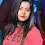## Sunday, December 2, 2012

### C/C++ Array Size and Length

Introduction

A simple note of array size and length test

Code

array_size_and_length.cpp

``````#include <iostream>

using namespace std;

/** Test basic array operation,
* the simple basic array, not std::array
*
*/
class TestClass {
public:
int _a;
double _b;
TestClass ()
{
_a = 1;
_b = 1.1;
}
TestClass (int a, double b) : _a(a), _b(b)
{
}
};
int getLength(int nums[]);
int getLength(TestClass vals[]);
int main() {
// an int array contains 3 elements
int nums[] = {1, 2, 5};
// TestClass array contains 2 elements
TestClass vals[] = {TestClass(), TestClass(2, 2.2)};
// output: total bytes of nums[]:                   12
cout << "total bytes of nums[]: \t\t\t" << sizeof(nums) << endl;
// output: element size of nums:                    4
cout << "element size of nums: \t\t\t" << sizeof(nums) << endl;
// output: number of element (length) of nums[]:    3
// this will work since sizeof(nums) is correct here
cout << "number of element (length) in nums[]: \t" << sizeof(nums)/sizeof(nums) << endl;
//
// output: wrong length of nums[]:                  1 (wrong value)
//
// Note: this will not work since only the address of nums (a pointer)
//       will be passed to getLength(int nums[]) function
cout << "wrong length of nums[]: \t\t" << getLength(nums) << " (wrong value)" << endl;
cout << endl;

// output: total bytes of vals[]:                   32
cout << "total bytes of vals[]: \t\t\t" << sizeof(vals) << endl;
// output: element size of vals:                    16
cout << "element size of vals: \t\t\t" << sizeof(vals) << endl;
// output: number of element (length) of vals[]:    2
// this will work since sizeof(vals) is correct here
cout << "number of element (length) in vals[]: \t" << sizeof(vals)/sizeof(vals) << endl;
//
// output: wrong length of vals[]:                  0 (wrong value)
//
// Note: this will not work since only the address of vals (a pointer)
//       will be passed to getLength(TestClass vals[]) function
cout << "wrong length of vals[]: \t\t" << getLength(vals) << " (wrong value)" << endl;
cout << endl;

system("PAUSE");
return 0;
}
int getLength(int nums[]) {
// sizeof(nums) -> 4, (pointer's size)
// sizeof(nums) -> 4, (size of int)
// return value is 1

return sizeof(nums)/sizeof(nums);
}
int getLength(TestClass vals[]) {
// sizeof(vals) -> 4, (pointer's size)
// sizeof(vals) -> 16, (size of TestClass)
// return value is 0

return sizeof(vals)/sizeof(vals);
}``````

Result

Reference

http://www.cplusplus.com/doc/tutorial/arrays/

1.